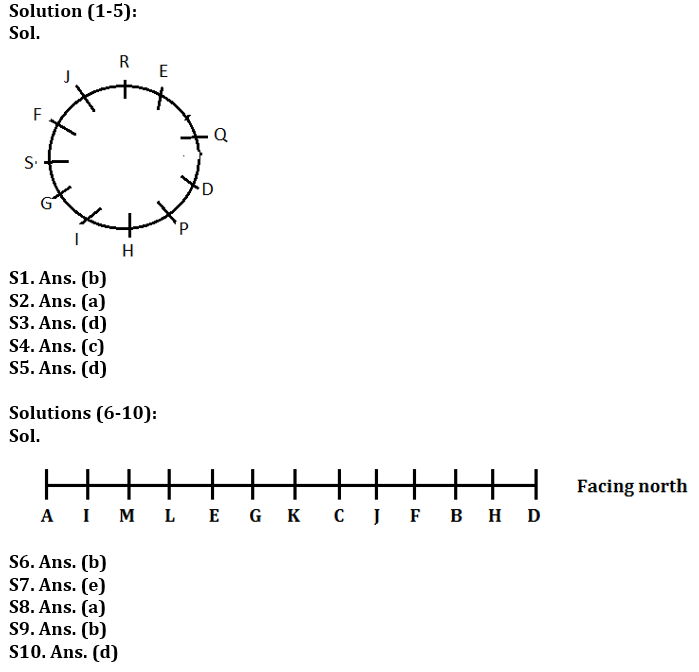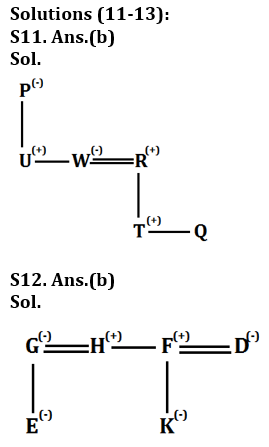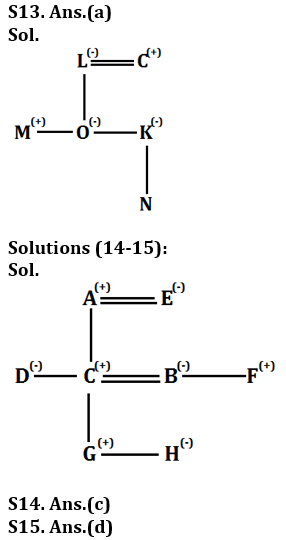Latest Banking jobs   »

# Reasoning Ability Quiz For Bank Foundation 2023-10th March

Directions (1-5): Study the following information carefully to answer the given questions
In a hostel, eleven students D, E, F, G, H, I, J, P, Q, R and S have decided to have dinner together at 9 PM, so they are sitting around a circular table facing the centre, but not necessarily in the same order. D sits second to the right of H who is not neighbour of J. Q is the immediate neighbour of D and E, and sits third to the left of J. G, who is on the immediate left of I is second to the right of F. S is on the immediate left of G and third to the right of R.

Q1. Who among the following is fourth to the right of J?
(a) S
(b) I
(c) D
(d) P
(e) None of these

Q2. Who among the following sits exactly between Q and P?
(a) D
(b) E
(c) H
(d) F
(e) None of these

Q3. How many persons are there sits between H and F?
(a) One
(b) Two
(c) None
(d) Three
(e) Four

Q4. Who among the following is third to the left of G?
(a) Q
(b) E
(c) J
(d) F
(e) None of these

Q5. Who among the following is an immediate neighbour of P?
(a) H
(b) D
(c) E
(d) Both H and D
(e) None of these

Directions (6-10): Study the information carefully and answer the questions given below.
Certain persons are sitting in a row. G is 2nd left to C. More than eight persons sit between A and H. M sits 3rd from one of the ends and immediate right to I. No one sits to the right of D. All the persons are facing same direction. F is not next to M. A is 4th left to E who is exactly between I and C. More than two persons between C and M. Three persons sit between J and D, who faces north. Five persons sit between I and C. M is second to the right of A. J sits immediate right to C. H sits 2nd to the right of F. L sits third left to K. B is one of the persons.

Q6. How many persons are sitting in the row?
(a) 12
(b) 13
(c) 14
(d) 15
(e) None of these

Q7. Who among the following sits 2nd to the right of K?
(a) E
(b) F
(c) C
(d) B
(e) None of these

Q8. What is the position of M with respect to G?
(a) 3rd to the left
(b) 3rd to the right
(c) 4th to the left
(d) 5th to the right
(e) None of these

Q9. How many persons sit to the right of H?
(a) Two
(b) One
(c) Three
(d) Four
(e) None of these

Q10. Who among the following sits to the immediate right of F?
(a) E
(b) A
(c) C
(d) B
(e) None of these

Directions (11-13): Each of these questions is based on the following information:
(i) A # B means A is the father of B.
(ii) A @ B means A is the mother of B.
(iii) A & B means A is the daughter of B.
(iv) A \$ B means A is the brother of B.
(v) A % B means A is the wife of B.

Q11. If the expression “P@U\$W%R#T\$Q” is true, then who among the following is uncle of Q?
(a) P
(b) U
(c) W
(d) R
(e) None of these

Q12. If the expression “E&G%H\$F#K&D” is true, then which of the following is not true?
(a) E is cousin of K
(b) F is aunt of E
(c) K is niece of H
(d) D is sister-in-law of H
(e) All are true

Q13. If the expression “M\$O&L%C#K@N” is true, then who among the following is grandmother of N?
(a) L
(b) O
(c) C
(d) M
(e) None of these

Directions (14-15): Study the following information carefully and answer the question given below-
There are eight persons in the family with two married couple. A is father of C. B is sister of F. E is mother of D. F is uncle of H. G is grandson of A. D is sister-in-law of B. C is father of H who is granddaughter of E. D is unmarried. B has two children.

Q14. Who among the following brother-in-law of C?
(a) A
(b) E
(c) F
(d) G
(e) None of these

Q15. Who among the following daughter-in-law of A?
(a) H
(b) F
(c) G
(d) B
(e) None of these

Solutions## FAQs

### What is the selection process of the Bank Clerk?

The selection process of the Bank Clerk is Prelims & Mains.

#### Congratulations!Union Budget 2023-24: Free PDF